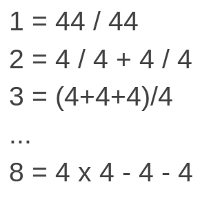# Arithmetic Puzzle - Make Numbers

### Make as many numbers as you can using just four digits!

By

There is a simple arithmetic puzzle, where you are given four digits, and you are told to build as many numbers as possible using just those four digits and the basic operations. For younger kids, that means plus, minus, divide and times, but as a child grows in their mathematical knowledge, operations such as powers, square roots, factorials and more might be used.

As I was saying, the classic version of this puzzle uses four 4's as the digits. As a child solves the puzzle, they might come up with

• 1 = 44 / 44
• 2 = 4 / 4 + 4 / 4
• 3 = (4+4+4)/4
• ...
• 8 = 4 x 4 - 4 - 4
• ...
Unfortunately, if you can only use the four basic operations and these four 4's, there's only 87 different numbers you can make, and about half of those are negative. Even 11 can't be done.

I've used a computer to figure out which sets of four digits make this arithmetic puzzle more interesting - which ones allow you to make the most numbers. This way, you can give puzzles like this to young schoolchildren, knowing that the puzzles are as interesting as they possibly can be.

The following worksheets list all the numbers from 0 to 100. For each number you'll see either something like

• 43 =
or something like
• 43 can't be done
. In the first case, it means that the puzzler should try to build the number 43 out of the digits given. In the second case, it means the number 43 can not be made out of the given digits using only addition, subtraction, multiplication and division.

There are many good ways to use these worksheets. For example, you could set them as a challenge puzzle in a classroom, perhaps with a prize for the most complete worksheet. Or, if you are a parent, you could just present the worksheets to the child as a fun puzzle.

As the child makes numbers from the given digits, they will be gaining numerous useful mathematical skills, including

• An intuitive sense of number, as they learn how numbers are related to each other,
• A sense of the arithmetic operations, as they see how numbers are transformed by them,
• Problem solving skills, as they explore to find 'just the right' formula for the more difficult numbers.

It's up to you whether you allow calculators to be used - personally I see no harm. A calculator will help eliminate frustrating arithmetic mistakes, but will not remove the need for creativity when solving the puzzles.

Anyway, here are the worksheets. Note that the solutions for some numbers require you to use double-digit numbers!

• For digits 1, 2, 7 and 8 (you can make 95 of the numbers from 0 to 100 with these digits)
• For digits 1, 3, 7 and 9 (you can make 93 numbers)
• For digits 2, 3, 4 and 7 (94 numbers)
• For digits 2, 3, 4 and 9 (93 numbers)
• For digits 2, 3, 5 and 8 (93 numbers)
• For digits 2, 3, 7 and 8 (93 numbers)
• For digits 2, 3, 8 and 9 (95 numbers)
• For digits 2, 4, 5 and 7 (93 numbers)
• For digits 2, 4, 8 and 9 (93 numbers)
• For digits 3, 4, 6 and 8 (93 numbers)
• For digits 3, 4, 7 and 9 (93 numbers)
• For digits 3, 6, 8 and 9 (94 numbers)

Yours, Dr Mike...